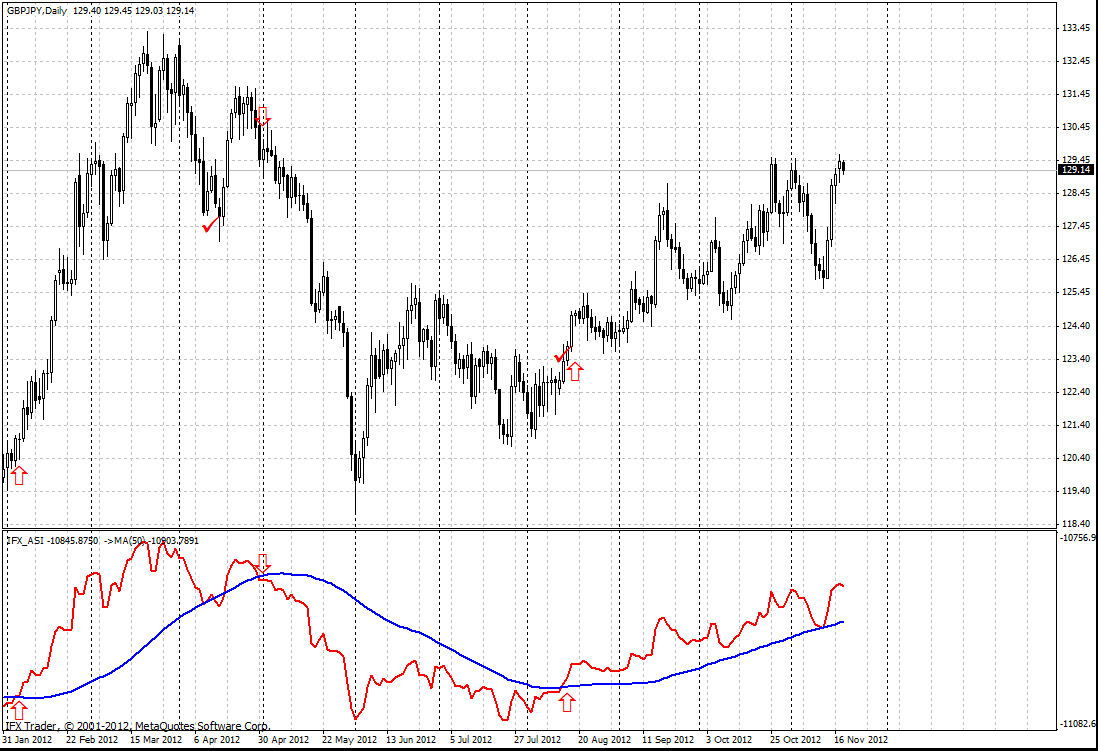#### Formula

SI(i)=50*(CLOSE(i-1)-CLOSE(i)+0,5*(CLOSE(i-1)-OPEN(i-1))+0,25*(CLOSE(i)-OPEN(i))/R)*(K/T)

ASI(i) = ASI(i-1) + SI(i), where

SI (i) - current value of Swing Index technical indicator;

SI (i - 1) - value of Swing Index technical indicator on the previous bar;

CLOSE (i) - current closing price;

CLOSE (i - 1) - previous closing price;

OPEN (i) - current opening price;

OPEN (i - 1) - previous closing price;

R - parameter which is calculated according to formula based on the ratio between current closing price and previous maximum and minimum.

K - is the biggest of two values: HIGH (i - 1) - CLOSE (i)) и (LOW (i - 1) - CLOSE (i));

T - maximum price change during trading session;

ASI (i) - current value of Accumulation Swing Index.